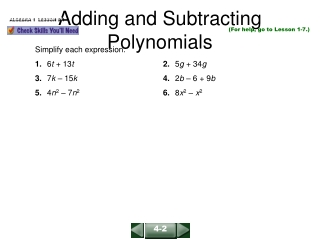DownloadDownload PresentationTélécharger la présentation- - - - - - - - - - - - - - - - - - - - - - - - - - - E N D - - - - - - - - - - - - - - - - - - - - - - - - - - -
Presentation Transcript

1. Adding and Subtracting Polynomials ALGEBRA 1 LESSON 9-1 (For help, go to Lesson 1-7.) Simplify each expression. 1. 6t + 13t2. 5g + 34g 3. 7k – 15k4. 2b – 6 + 9b 5. 4n2 – 7n26. 8x2 – x2 4-2

2. Adding and Subtracting Polynomials ALGEBRA 1 LESSON 9-1 Solutions 1. 6t + 13t = (6 + 13)t = 19t 2. 5g + 34g = (5 + 34)g = 39g 3. 7k – 15k = (7 – 15)k = –8k4. 2b – 6 + 9b = (2 + 9)b – 6 = 11b – 6 5. 4n2 – 7n2 = (4 – 7)n2 = –3n26. 8x2 – x2 = (8 – 1)x2 = 7x2 4-2

3. Degree: 0 The degree of a nonzero constant is 0. Degree: 4 The exponents are 1 and 3. Their sum is 4. Degree: 1 6c = 6c1. The exponent is 1. Adding and Subtracting Polynomials ALGEBRA 1 LESSON 9-1 Find the degree of each monomial. a. 18 b. 3xy3 c. 6c 4-2

4. Place terms in order. 7x – 2 3x5 – 2x5 + 7x – 2 Place terms in order. x5 + 7x – 2 Combine like terms. Adding and Subtracting Polynomials ALGEBRA 1 LESSON 9-1 Write each polynomial in standard form. Then name each polynomial by its degree and the number of its terms. a. –2 + 7x linear binomial b. 3x5 – 2 – 2x5 + 7x fifth degree trinomial 4-2

5. 6x2 + 3x + 7 2x2 – 6x – 4 8x2 – 3x + 3 Group like terms. Then add the coefficients. (6x2 + 3x + 7) + (2x2 – 6x – 4) = (6x2 + 2x2) + (3x – 6x) + (7 – 4) Adding and Subtracting Polynomials ALGEBRA 1 LESSON 9-1 Simplify (6x2 + 3x + 7) + (2x2 – 6x – 4). Method 1:Add vertically. Line up like terms. Then add the coefficients. Method 2:Add horizontally. = 8x2 – 3x + 3 4-2

6. (2x3 + 4x2 – 6) Line up like terms. –(5x3 – 2x – 2) 2x3 + 4x2 – 6 Add the opposite. –5x3– 2x+ 2 –3x3 + 4x2 – 2x – 4 Adding and Subtracting Polynomials ALGEBRA 1 LESSON 9-1 Simplify (2x3 + 4x2 – 6) – (5x3 + 2x – 2). Method 1:Subtract vertically. Line up like terms. Then add the coefficients. 4-2

7. = (2x3 – 5x3) + 4x2 – 2x + (–6 + 2)Group like terms. = –3x3 + 4x2 – 2x – 4 Simplify. Adding and Subtracting Polynomials ALGEBRA 1 LESSON 9-1 (continued) Method 2: Subtract horizontally. (2x3 + 4x2 – 6) – (5x3 + 2x – 2) = 2x3 + 4x2 – 6 – 5x3– 2x+ 2 Write the opposite of each term in the polynomial being subtracted. 4-2

8. Adding and Subtracting Polynomials ALGEBRA 1 LESSON 9-1 Simplify each expression. Then name each polynomial by its degree and number of terms. 1. –4 + 3x – 2x2 2. 2b2 – 4b3 + 6 3. (2x4 + 3x – 4) + (–3x + 4 + x4) 4. (–3r + 4r2 – 3) – (4r2 + 6r – 2) –2x2 + 3x – 4; quadratic trinomial –4b3 + 2b2 + 6; cubic trinomial 3x4; fourth degree monomial –9r – 1; linear binomial 4-2